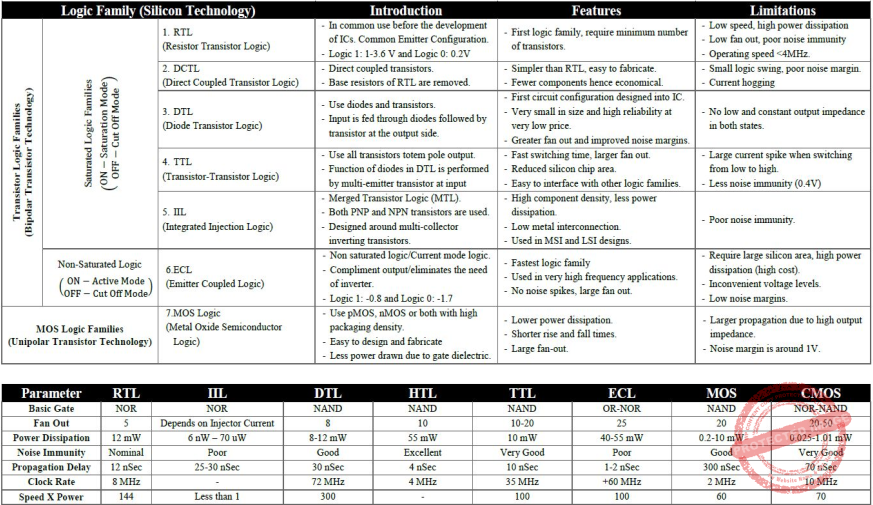## Logic-Families

 Question 1
The following diagram depicts ____ logicA Diode B Transistor C Diode transistor D Resistor
Digital-Logic-Design       Logic-Families       KVS DEC-2013
Question 1 Explanation:
The given diagram is combination of both Diode and Transistor
 Question 2
Based on the current technology,___ is the fastest logic family
 A CMOS B TTL C MOS D ECL
Digital-Logic-Design       Logic-Families       KVS DEC-2017
Question 2 Explanation:Question 3
When used with IC, what does he term QUAD indicate?
 A 2 circuits B 4 circuits C 6 circuits D 8 circuits
Digital-Logic-Design       Logic-Families       KVS 30-12-2018 Part B
Question 3 Explanation:
→A quad gate is an IC (integrated circuit or chip) containing four logic gates. The gates can be of any type: AND, OR, XOR, NOT, NAND, NOR, and XNOR. Within any given quad gate, all four of the individual gates are normally of the same type.
→A logic gate is an elementary building block of a digital circuit.
 Question 4
When a tri - state logic device is in the third state, then :
 A it draws low current B it does not draw any current C it draws very high current D it presents a low impedance
Digital-Logic-Design       Logic-Families       UGC NET CS 2004 Dec-Paper-2
Question 4 Explanation:
Three Stage logic name itself implies there are 3 states of the buffer.
1. High (1)
2. Low (0)
3. High Impedance (Z)
→ High impedance means that a point in a circuit (a node) allows a relatively small amount of current through, per unit of applied voltage at that point.
→ High impedance circuits are low current, high voltage, whereas low impedance circuits are the opposite.
Truth table of Tri-state logic InverterThere are 4 questions to complete.

Register Now